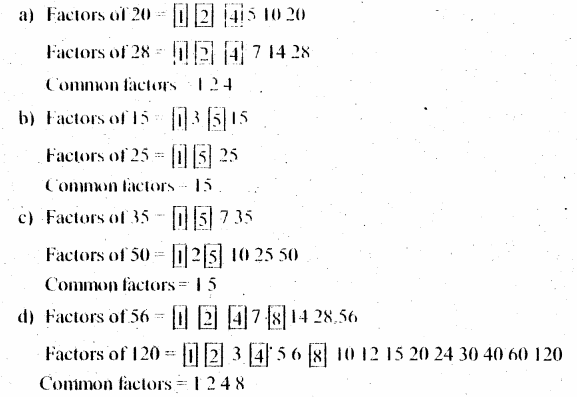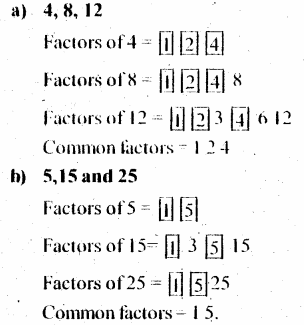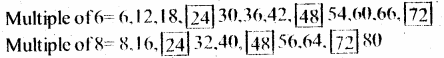# KSEEB Solutions for Class 6 Maths Chapter 3 Playing with Numbers Ex 3.4

Students can Download Chapter 3 Playing with Numbers Ex 3.4 Questions and Answers, Notes Pdf, KSEEB Solutions for Class 6 Maths helps you to revise the complete Karnataka State Board Syllabus and score more marks in your examinations.

## Karnataka State Syllabus Class 6 Maths Chapter 3 Playing with Numbers Ex 3.4

Question 1.
Find the common factors of:
a) 20 and 28
b) 15 and 25
c) 35 and 50
d) 56 and 120
Solution:Question 2.
Find the common factors of
a) 4, 8 and 12
b) 5, 15 and 25
Solution:Question 3.
Find first three common multiples of:
a) 6 and 8
b) 12 and 18
Solution:
a) 6 and 8:-3 common multiples = 24, 48, 72

b) 12 and 18:-
Multiples of 12 = 12, 24, 36, 48, 60, 72
Multiples of 18= 18, 36, 54, 72
3 Common multiples = 36, 72, 108

Question 4.
Write all the number less than 10(1 which are common multiples of 3 and 4
Solution:
Multiples of 3 = 3, 6, 0, 12, 15, 18, 21, 24, 27, 30
Multiples of 4 = 4, 8, 12, 16, 20, 24, 28, 32, 36, 40
Common multiples = 12, 24, 36, 48, 60, 72, 84, 96Question 5.
Which of the following numbers are co-prime?
a) 18 and 35
b) 15 and 37
c) 30 and 415
d) 17 and 68
e) 216 and 215
f) 81 and 16
Solution:
a) Factors of 18 = 1, 2, 3, 6, 9, 18
Factors of 35 = 1, 5, 7, 35
Common factors = 1
Therefore, the given two numbers are co-prime

b) Factors of 15 = 1, 3, 5, 15
Factors of 37 = 1, 37
Common factors = 1
The given two numbers are co-prime

c) Factors of 30 = 1, 2, 3, 5, 6, 10, 15, 30
Factors of 415 = 1, 5, 83, 415
Common factors = 1, 5
As these numbers have a common factors other than 1, the given two numbers arc not co-prime

d) Factors of 17 = 1, 17
Factors of 68 = 1, 2, 4, 17, 34, 68
Common factors = 1, 17
As these numbers have a common factors other than 1, the given two numbers are not co-primee) Factors of 216 = 1, 2, 3, 4, 6, 8, 9, 12, 18, 24, 17, 36, 54, 72, 108, 216
Factors of 215 = 1, 5, 43, 215
Common factors = 1
The given two numbers are prime.

f) Factors of 81= 1, 3, 9, 27, 81
Factors of 16 = 1, 2, 4, 8, 16
Common factors = 1
Therefore the given two numbers are co-prime.

Question 6.
A number is divisible by both 5 and 12. By which other number will that number be always divisible?
Solution:
Factors of 5 = 1, 5
Factors of 12 = 1, 2, 3, 4, 5, 6, 12
As the common factors of these number is 1, the given two numbers are co-prime and the number will also be divisible by their product , i,e 60 and the factors of 60.
i.e, 1, 2, 3, 4, 5, 6, 10, 12, 15, 20, 30, 60.Question 7.
A number is divisible by 12. By what other numbers will that number be divisible?
Solution:
Since, the number is divisible by 12, it will also be divisible 1,2,3,4 and 6 are numbers other than 12 by which this number is also divisible.